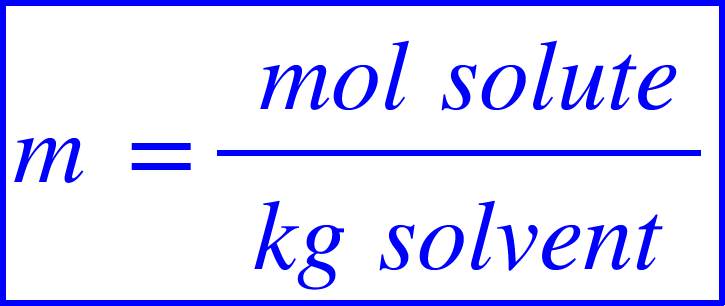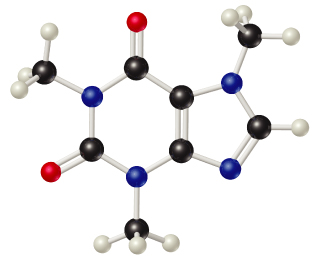# Problem: Caffeine (C8H10N4O2) is a stimulant found in coffee and tea. A solution of caffeine in the solvent chloroform (CHCl3) has a concentration of 0.0510 m.Calculate the percentage of caffeine by mass in the solution.

###### FREE Expert Solution

Molality of a solution is defined as the number of moles of solute per kilogram of solvent. It is represented as:We will solve this problem through these steps:

• Find the molar mass of caffeine
• Find the number of moles of caffeine and mass of chloroform using the molality of solution
• Find the mass of caffeine
• Find the mass percent of caffeine is solution

90% (336 ratings)###### Problem Details
Caffeine (C8H10N4O2) is a stimulant found in coffee and tea. A solution of caffeine in the solvent chloroform (CHCl3) has a concentration of 0.0510 m.Calculate the percentage of caffeine by mass in the solution.